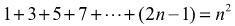##### Algebra II All-in-One For Dummies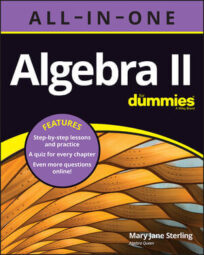Here it is. You have this All-in-One reference for concepts and formulas occurring in Algebra II. The material here is grouped by general algebraic content to make it easier to find what you need. The formulas have the standard mathematical format with variables appearing as x, y, and z and the constant numbers appearing as letters at the beginning of the alphabet.

## Line formulas

When graphing segments and lines containing points on the coordinate plane, you have the opportunity to find many values: slope, distance, midpoint, and so on. When using these formulas, you see the coordinates of points written in (xa, ya) format. Note that the slope of a line is designated with a small m and the midpoint of a segment with a capital M.

Formulas to use when given the points (x1,y1) and (x2,y2).

Slope of line through the points: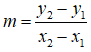Midpoint of the segment between the points: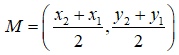Distance between the points: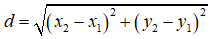Given the equation in slope-intercept form: y = mx + b

Slope of a parallel line: m

Slope of a perpendicular line: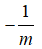Given the standard form of a line: Ax + By = C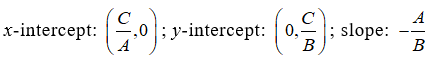## Miscellaneous formulas

When you can’t find a particular formula under any of the other headings, this is where you look. There’s a little geometry, some counting rules, and formats for basic factoring.

Distance:

d = rt, where r is the rate of speed and t is the time in that same rate.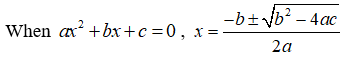Factorial:

n! = n ∙ (n – 1) ∙ (n – 2) ∙ (n – 3) ∙∙∙ 3 ∙ 2 ∙ 1, where n is a non-negative integer.

Special rule: 0! = 1

Absolute value: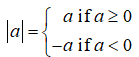Factoring:

Difference of squares:

a² – b² = (ab)(a + b)

Difference of cubes:

a³ – b³ = (ab)(a² + ab + b²)

Sum of cubes:

a³ + b³ = (a + b)(a² – ab + b²)

Counting:

Multiplication property: m1m2m3 ∙∙∙, where event 1 can happen m1 ways, event 2 can happen m2 ways, and so on.

Permutations: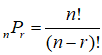, where n is the total number of ways an event can happen and r is the selected number.

Combinations: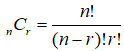, where n is the total number of ways an event can happen and r is the selected number.

Binomial Theorem: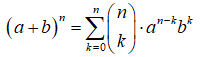Heron’s formula: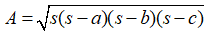## Inequality equivalences

When solving absolute value inequalities, you need to change the format so you can perform the usual algebraic processes and solve for the value of the variable.

(This applies to < and > also):

For | ax + b | ≤ c, solve –cax + bc .

For | ax + b | ≥ c, solve ax + b ≤ –c and ax + bc .

## Proportions

A proportion is an equation involving two ratios. Changing the initial format of a proportion can be most helpful.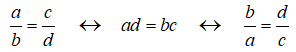## Conics standard equations

In the case of the four basic conic sections, you have the consistent property that the center or vertex of the conic is (h,k).

Parabola: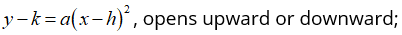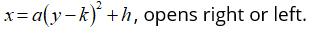Circle:

(xh)2 + (yk)2 = r2 , where r is the radius.

Ellipse: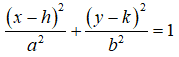Hyperbola: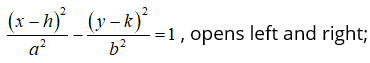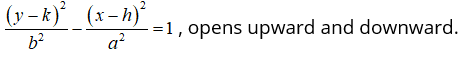## Exponential and logarithmic equations and functions

Exponential and logarithmic functions have some special properties that allow you to simplify expressions and move from one format to another.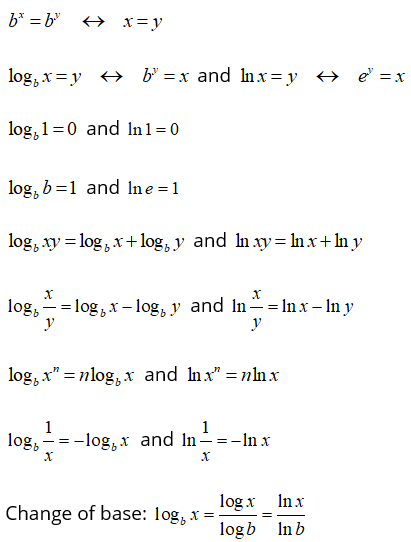## Cramer's Rule

Systems of linear equations can be solved using the standard algebraic processes. But Cramer’s Rule is a wonderful option when the solutions involve large and un-factorable coefficients resulting in complicated fractions.

The solution of the system of linear equations: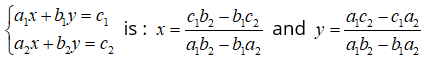## Properties

The basic properties of algebraic expressions allow you to make adjustments without changing the value of the expression. An alternate format is often more desirable.

 Property Math Statement Commutative (of addition) a + b = b + a Commutative (of multiplication) a ∙ b = b ∙ a Associative (of addition) a + (b+c) = (a+b) + c Associative (of multiplication) a (b ∙ c) = (a ∙ b) c Distributive (multiplication over addition) a (b+c) = a ∙ b + a ∙ c Distributive (multiplication over subtraction) a (b–c) = a ∙ b – a ∙ c Identity (of addition) a + 0 = 0 + a = a Identity (of multiplication) a ∙ 1 = 1 ∙ a = a Multiplication property of zero a ∙ b ∙ c ∙ d ∙ e ∙ f = 0 → a, b, c, d, e or f = 0 Additive inverse a + (–a) = 0 Multiplicative inverse a ∙ (1/a) = 1, a ≠ 0

## Pascal's Triangle

When raising the binomial (a + b)n to a desired power, you can quickly perform the expansion using Pascal’s Triangle to help create the coefficient of each term in the expansion.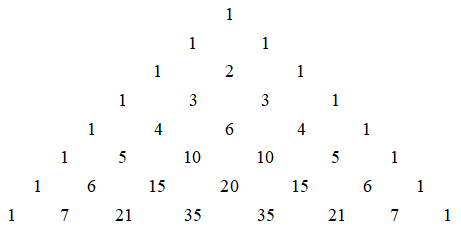## Function transformations in graphing

The basic graphs of lines, parabolas, trig functions, and so on are transformed with stretches, flattening, reflections, and translations around the grid. The formulas here represent the transformation of a function ƒ(x) using the constants h and a.

Translations:

Translating up: ƒ(x) + h

Translating down: ƒ(x) – h

Translating right: ƒ(xh)

Translating left: ƒ(x + h)

Reflections:

Reflecting over the x-axis: –ƒ(x)

Reflecting over the y-axis: ƒ(–x)

Scaling:

Stretching (steepening):  a ∙ ƒ(x) when a > 1

Compressing (flattening): a ∙ ƒ(x) when 0 < a < 1

## Sum of series

A series is the sum of terms in a sequence. The sum can be some of the terms or all of them. Use the formulas rather than listing the terms and adding them together.

Arithmetic: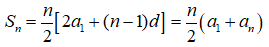Geometric: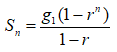First n squares of the positive integers: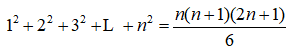First n cubes of the positive integers: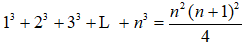First n odd positive integers: